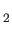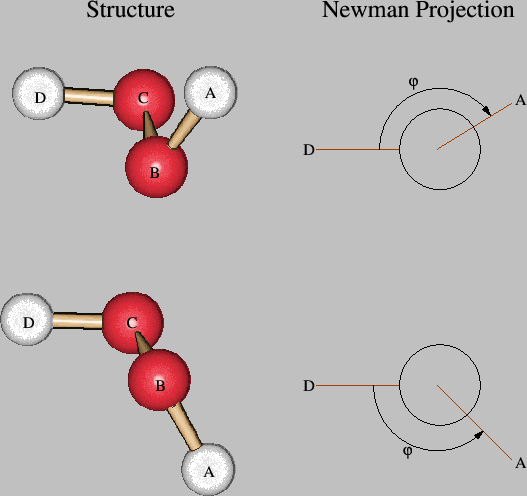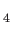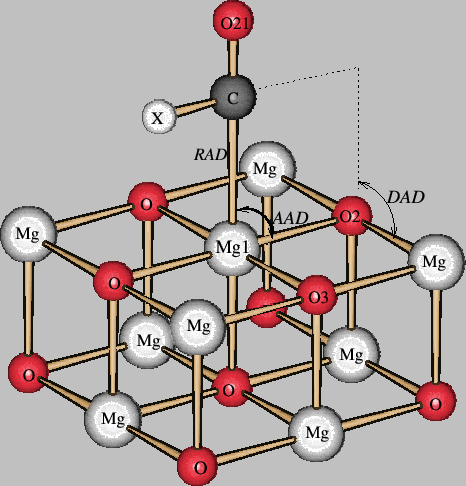### Keyword GEOMETRY

This keyword is mandatory! It specifies the molecular geometry.
Options:
CARTESIAN / ZMATRIX / MIXED
 CARTESIAN The molecular structure is given in Cartesian coordinates. ZMATRIX The molecular structure is defined by a Z-matrix. The form Z-MATRIX is equivalent to ZMATRIX. MIXED The first atoms of the molecular structure are defined by Cartesian coordinates and the following ones are defined by a Z-matrix.
ANGSTROM / BOHR
 ANGSTROM Coordinates or bond distances are given in Ångströms. BOHR Coordinates or bond distances are given in atomic units.
Description:
The geometry is read in the keyword body of GEOMETRY in free format, one line for each atom. In the case of a CARTESIAN input the atomic symbol (e.g. H), which may carry an identification number (e.g. H1), and the x, y and z coordinates of each atom of the system must be specified. As an example, the geometry of HO may be specified as:

```
GEOMETRY CARTESIAN ANGSTROM
O  0.00  0.00  0.00
H  0.76  0.00  0.52
H -0.76  0.00  0.52
```

The Cartesian coordinates of an individual atom, defined by the atomic symbol (e.g. H2), or an atom group, defined by the element symbol (e.g. H), can be frozen during the geometry optimization in redundant coordinates. To keep all three degrees of freedom of an atom fixed the corresponding atomic symbol or element symbol has to be specified with the string XYZ in the keyword body of CONSTANTS. The string X will freeze only the x coordinate of the corresponding atom, the string XY fixes the x and y coordinates, etc. In the following example, the oxygen atom is kept frozen and the movements of all hydrogen atoms are restricted to the xz-plane (y coordinate frozen) during the optimization.

```
GEOMETRY CARTESIAN ANGSTROM
O  0.00  0.00  0.00
H  0.76  0.00  0.52
H -0.76  0.00  0.52
#
CONSTANTS
O XYZ
H Y
```

As usual, the comment line (#) is given only for clarity. The effective nuclear charge (in atomic units), which is identical to the number of electrons counted for the atom, and the nuclear mass (in atomic mass units; amu) can be specified by an integer and real number after the coordinates, respectively. Therefore, the following input for a water-like system consists of a frozen oxygen atom, a hydrogen atom, H1, fixed in the xz-plane with the effective nuclear charge 0, i.e. a ghost atom that can be used to define basis functions at a point in space, and another hydrogen atom, H2, with the nuclear charge 1 and the nuclear mass 2.014, i.e. a deuterium atom.

```
GEOMETRY CARTESIAN ANGSTROM
O   0.00  0.00  0.00
H1  0.76  0.00  0.52  0
H2 -0.76  0.00  0.52  1  2.014
#
CONSTANTS
O  XYZ
H1 Y
```

If quantities are not specified, default values are used (e.g. the O atom in the example above has a nuclear charge of 8 and a mass of 15.999 amu).

Negative integer numbers after the atomic coordinates indicate an MM atom. The absolute value of this integer number specifies the atom type in the selected force field. These atom types can be found in the FFDS file. Thus, the geometry part of an MM water input can have the form:

```
GEOMETRY CARTESIAN ANGSTROM
O   0.00  0.00  0.00  -76
H1  0.76  0.00  0.52  -77
H2 -0.76  0.00  0.52  -77
```

Here 76 and 77 are the atom types of the MM oxygen and hydrogen atoms, respectively. Note that the connectivity between the atoms is generated automatically. The following input shows the geometry definition of a QM/MM water dimer, the first one being QM and the second one being MM.

```
GEOMETRY CARTESIAN ANGSTROM
O     1.159094    0.359472   -1.228183     8  15.999400
H     0.165274    0.461227   -1.247218     1   1.007940
H     1.357609   -0.118689   -2.058595     1   1.007940
O    -1.496793    0.620303   -1.289943    -76
H    -2.123457    0.067624   -0.732608    -77
H    -1.996365    1.430082   -1.611984    -77
```

For the QM atoms the nuclear charges and masses are also explicitly defined in this input. Again the connectivity of the MM atoms is generated automatically.

Even though it is usually advantageous to sort a QM/MM geometry definition according to the QM and MM atoms, this is not mandatory for a deMon2k input. In the following input QM and MM atoms are mixed among themselves. Note also the extended element symbols. This naming must be chosen such that the first two characters remain specific for the element. Thus, Hm and Hq used below are possible choices for hydrogen whereas He or Ho would denote helium or holmium, respectively!

```
GEOMETRY CARTESIAN ANGSTROM
Hm1b  1.262389    -1.937796     1.222724   -77  6
Hq1a  0.217713     0.505507    -1.369657
Hm1a  1.124357    -0.596134     0.419304   -77  6
Hq1b  1.503324    -0.129952    -1.999029
Oq2  -1.628323     0.600651    -1.413703
Om1   0.867185    -1.043515     1.275905   -76  1  3
Hq2a -1.885969     0.153697    -0.557057
Om2  -1.948708    -0.776469     1.058175   -76  10 12
Hq2b -2.021673     1.495719    -1.360149
Hm2a -0.978710    -0.948944     1.231841   -77  8
Oq1   1.187632     0.332759    -1.195914
Hm2b -2.264749    -0.314968     1.861890   -77  8
```

In this input the integer numbers after the negative atom type specifications of the MM atoms denote their connectivity. Thus, hydrogen atom Hm1b and Hm1a are connected to atom 6 which is the MM oxygen atom Om1. For this atom two connectivity integer numbers are given, 1 and 3, that indicate the connectivities to atom 1, Hm1b, and atom 3, Hm1a.

If the geometry of the system is given by a Z-matrix, using the option ZMATRIX, each line in the keyword body of GEOMETRY describes the connectivity of the atom, which again is defined by its atomic symbol. The first atom (A) of the Z-matrix defines the origin of the input (see Figure 2) and therefore has no connectivity information.

Figure 2: Orientation of the first three atoms defined in the Z-matrix.The second atom (B) lies on the z-axis of the input coordinate system and is connected to the first atom at a distance RAB. The third atom (C) lies in the xz-plane and connects to the two previously defined atoms at a distance RBC and an angle TABC. Each of the following atoms is defined by an input line in free format containing the information:

LABEL NB RAB NC TABC ND PABCD

Here LABEL is the atomic label of atom A. NB, NC, and ND are the Z-matrix line numbers or the atomic labels of the atoms B, C and D with respect to which atom A is defined by a length (RAB), angle (TABC), and dihedral angle (PABCD). These are the so-called internal coordinates of the system. Their values are given by RAB (in Ångström or Bohr), TABC, and PABCD (in degrees), respectively. The maximum length of these numbers in the Z-matrix is limited to ten characters. The dihedral angle is defined by the angle between the planes spanned by the atoms {A, B, C} and {B, C, D}, respectively. The sign of the dihedral angle is defined according to the Newman projection shown in Figure 3. If the projection angle is oriented clockwise then the dihedral angle is positive.

Figure 3: Definition of positive (top) and negative (bottom) dihedral angles in deMon2k.Instead of giving the values for RAB, TABC, and PABCD directly in the Z-matrix, they may also be represented by symbolic strings with no more than eight characters each. These strings must be assigned to CONSTANT or VARIABLE values (see 4.1.3 and 4.1.5) in the input, as the following example of the CHinput demonstrates:

```
GEOMETRY ZMATRIX ANGSTROM
C1
C2  C1  rCC
H1  C1  rCH  C2  aCCH
H2  C1  rCH  C2  aCCH  H1  dHCCH
H3  C2  rCH  C1  aCCH  H2  dHCCH
H4  C2  rCH  C1  aCCH  H1  dHCCH
#
VARIABLES
rCC   1.3390
rCH   1.0850
aCCH  121.09
#
CONSTANT
dHCCH 180.0
```

The internal coordinates listed under VARIABLES are changed during geometry optimization, whereas the coordinates listed under CONSTANTS are kept frozen. The RAB, TABC, and PABCD values that are directly defined in the Z-matrix are always interpreted as constants in internal or internal redundant structure optimizations.

If one uses internal coordinates, dummy atoms are often useful. The atomic symbol X has been reserved for them (note that the symbol XX is reserved for automatically generated dummy atoms; it is not to be processed as an input symbol). The following example shows the use of a dummy atom in the definition of HCN.

```
GEOMETRY ZMATRIX ANGSTROM
H
C  1  R1
X  2  1.0  1  90.0
N  2  R2   3  A1    1  180.0
#
VARIABLES
R1  1.089
R3  1.166
A1  90.00
```

Here the dummy atom is used to avoid a 180angle which might cause problems in a geometry optimization. Please note that the structure parameters which are defined inside the Z-matrix are kept constant during structure optimization. Exampleon pageof the deMon2k tutorial shows the use of a dummy atom for the definition of a ring system.

Table 4: Substitution strings for the internal coordinate substitution in the Z-matrix.
 Subst. String Internal Coordinates Description RAD RAB TABC PABCD No substitution. RRD RAB RAC PABCD Length AC substitutes for angle ABC. RAA RAB TABC TABD Angle ABD substitutes for dihedral ABCD. RRA RAB RAC TABD Combination of RRD and RAA. RRR RAB RAC RAD Definition over lengths only.

To facilitate the input of the internal coordinates, substitutions of angles and dihedral angles are possible in the Z-matrix input. The substitutions are indicated by strings after the connectivity information in each Z-matrix line. The possible substitution strings are listed in Table 4. Thus, the connectivity information in a Z-matrix line can be extended by the substitution string, the nuclear charge, and the atomic mass, respectively. This ordering is necessary! However, defaults can be omitted. That is, the definition of a substitution string and an atomic mass will work and the default nuclear charge will be assigned to the given atom. The examplesandon pageand pageof the tutorial show some common applications of Z-matrix inputs.

If the geometry of the system is given by a MIXED input, the first few atoms are defined by Cartesian coordinates. At least the first three atoms, including dummy atoms, must be defined in this way. Subsequent atoms can then be defined by a Z-matrix line as described above. The reference atoms in that Z-matrix line can be defined either by Cartesian or internal coordinates. In either case, the coordinate system orientation is defined by the first three atoms. The following example, depicted in Figure 4, shows a MIXED input for a CO molecule adsorbed on a MgO cluster.

Figure 4: Coordinate definition of the example MIXED input.```
GEOMETRY MIXED ANGSTROM
Mg1    0.000   0.000   0.000
O2     0.000   2.105   0.000
O3     2.105   0.000   0.000
O4     0.000  -2.105   0.000
O5    -2.105   0.000   0.000
Mg6    2.105   2.105   0.000
Mg7    2.105  -2.105   0.000
Mg8   -2.105  -2.105   0.000
Mg9   -2.105   2.105   0.000
O10    0.000   0.000  -2.105
Mg11   0.000   2.105  -2.105
Mg12   2.105   0.000  -2.105
Mg13   0.000  -2.105  -2.105
Mg14  -2.105   0.000  -2.105
O15    2.105   2.105  -2.105
O16    2.105  -2.105  -2.105
O17   -2.105  -2.105  -2.105
O18   -2.105   2.105  -2.105
X20    C19     1.0     Mg1     90.0     O2      180.0
O21    C19     RCO     X20     90.0     Mg1     180.0
#
CONSTANTS
Mg   XYZ
O2   XYZ
O3   XYZ
O4   XYZ
O5   XYZ
O10  XYZ
O15  XYZ
O16  XYZ
O17  XYZ
O18  XYZ
#
VARIABLES
RCO  1.4
```

This example calls for the free optimization of a CO molecule, including internal relaxation, on a frozen MgO cluster surface. Thus, the MgO cluster is kept constant by freezing its Cartesian coordinates (see 4.1.3). The carbon atom of the CO molecule is defined with respect to the Mg1, O2, and O3 atoms of the cluster (see Figure 4) through the internal coordinates RAD, AAD, and DAD. These coordinates are free for optimization because they are declared as variables (see 4.1.5). The same holds for the CO bond length that is defined by the internal coordinate RCO. The dummy atom X20 must be used to avoid an ill-defined dihedral angle if the O21, C, Mg1 arrangement becomes linear.

In deMon2k Z-MATRIX and MIXED geometry definitions are also available for MM and QM/MM calculations. Note that the dummy atom line in the following Z-matrix definition for an MM input has to be taken into account for the MM connectivity numbering.

```
GEOMETRY Z-MATRIX ANGSTROM
O                                        -76    15.999400 2 3
H    1  R1                               -77     1.007940 1
H    1  R2     2  A1                RAD  -77     1.007940 1
H    1  R3     2  A2     3  D1      RAD  -77     1.007940 6
X1   4  RCON   1  ACON   2  DCON    RAD    0     0.000000
O    4  R4     5  A3     1  D2      RAD  -76    15.999400 4 7
H    6  R5     4  A4     5  D3      RAD  -77     1.007940 6
#
VARIABLES
R1          1.00275988
R2          1.00278094
R3          1.72377877
R4          1.01622488
R5          0.99833459
A1          108.809982
A2          122.743681
A3           86.814590
A4          108.264348
D1         -152.130713
D2          181.980680
D3           33.280793
#
CONSTANTS
RCON        2.00000000
ACON         90.000000
DCON       -138.000000
```